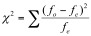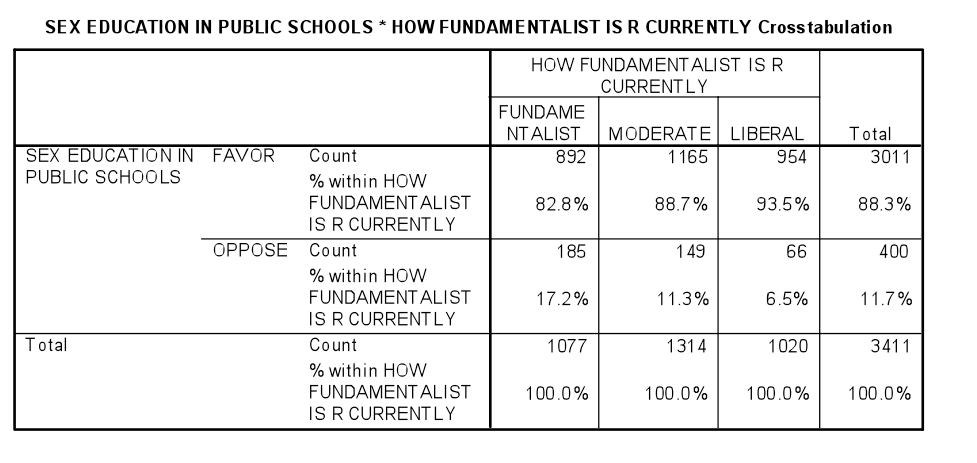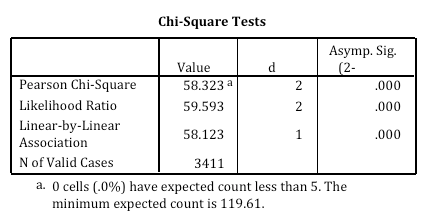The Chi Square statistic is commonly used for testing relationships between categorical variables.  The null hypothesis of the Chi-Square test is that no relationship exists on the categorical variables in the population; they are independent.  An example research question that could be answered using a Chi-Square analysis would be:

Is there a significant relationship between voter intent and political party membership?# Quantitative Results in One-HourScreen share with a statistician as we walk you through conducting and understanding your interpreted analysis. Have your results draft complete in one hour with guaranteed accuracy.

How does the Chi-Square statistic work?

The Chi-Square statistic is most commonly used to evaluate Tests of Independence when using a crosstabulation (also known as a bivariate table).  Crosstabulation presents the distributions of two categorical variables simultaneously, with the intersections of the categories of the variables appearing in the cells of the table.  The Test of Independence assesses whether an association exists between the two variables by comparing the observed pattern of responses in the cells to the pattern that would be expected if the variables were truly independent of each other.  Calculating the Chi-Square statistic and comparing it against a critical value from the Chi-Square distribution allows the researcher to assess whether the observed cell counts are significantly different from the expected cell counts.

The calculation of the Chi-Square statistic is quite straight-forward and intuitive:where fo = the observed frequency (the observed counts in the cells)
and fe = the expected frequency if NO relationship existed between the variables

As depicted in the formula, the Chi-Square statistic is based on the difference between what is actually observed in the data and what would be expected if there was truly no relationship between the variables.

How is the Chi-Square statistic run in SPSS and how is the output interpreted?

The Chi-Square statistic appears as an option when requesting a crosstabulation in SPSS. The output is labeled Chi-Square Tests; the Chi-Square statistic used in the Test of Independence is labeled Pearson Chi-Square. This statistic can be evaluated by comparing the actual value against a critical value found in a Chi-Square distribution (where degrees of freedom is calculated as # of rows – 1 x # of columns – 1), but it is easier to simply examine the p-value provided by SPSS. To make a conclusion about the hypothesis with 95% confidence, the value labeled Asymp. Sig. (which is the p-value of the Chi-Square statistic) should be less than .05 (which is the alpha level associated with a 95% confidence level).

Is the p-value (labeled Asymp. Sig.) less than .05?  If so, we can conclude that the variables are not independent of each other and that there is a statistical relationship between the categorical variables.In this example, there is an association between fundamentalism and views on teaching sex education in public schools.  While 17.2% of fundamentalists oppose teaching sex education, only 6.5% of liberals are opposed.  The p-value indicates that these variables are not independent of each other and that there is a statistically significant relationship between the categorical variables.

What are special concerns with regard to the Chi-Square statistic?

There are a number of important considerations when using the Chi-Square statistic to evaluate a crosstabulation.  Because of how the Chi-Square value is calculated, it is extremely sensitive to sample size – when the sample size is too large (~500), almost any small difference will appear statistically significant.  It is also sensitive to the distribution within the cells, and SPSS gives a warning message if cells have fewer than 5 cases. This can be addressed by always using categorical variables with a limited number of categories (e.g., by combining categories if necessary to produce a smaller table).

Statistics Solutions can assist with your quantitative analysis by assisting you to develop your methodology and results chapters. The services that we offer include:

Data Analysis Plan

• Edit your research questions and null/alternative hypotheses
• Write your data analysis plan; specify specific statistics to address the research questions, the assumptions of the statistics, and justify why they are the appropriate statistics; provide references
• Justify your sample size/power analysis, provide references
• Explain your data analysis plan to you so you are comfortable and confident